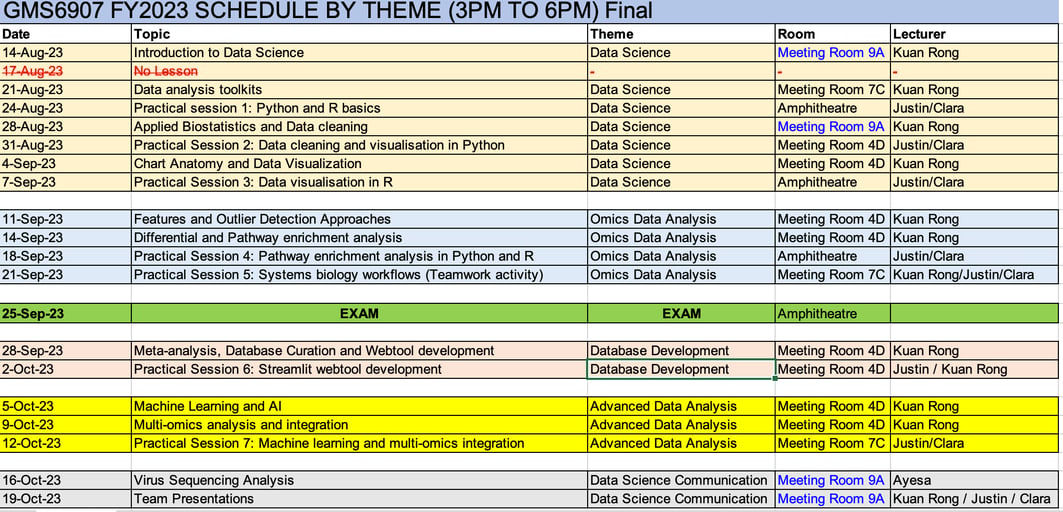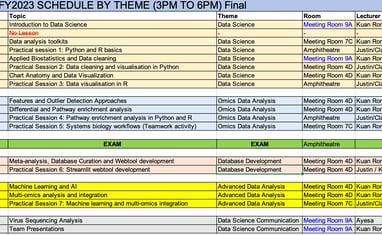### Timetable for FY2023 (3pm - 6pm)### Module assessment

Class participation and Attendance: 20%

Mid-term Exam: 40%

Team-based project presentation: 40%

Module details

Week 1 An Introduction to Data Science

Learning objectives

At the end of the lesson:

1. Students are expected to understand why data science is important at present and in the future.

2. Students should appreciate the changing computational biology landscape

3. Students should know the importance of reproducibility in data science and how to improve data reproducibility

4. Students should understand the best practices for file naming

5. Students should be able to identify different types of data and strategise the best methods to use for interpreting different data types.

Learning objectives

At the end of the lesson:

1. Students should be able to understand the strengths and limitations of Microsoft Excel

2. Students should know how to use Excel functions, including but not limited to Excel formulas, filter functions, sort and filter functions

3. Students should be able to know the most common errors inherent with Excel analysis.

4. Students should know how to plot publication quality figures and panels with Graphpad Prism

Week 2 GitHub and Introduction to Python

Learning objectives

At the end of the lesson:

1. Students should know how to naviage GitHub and understand some of the useful features of the GitHub repository

2. Students should understand simple markdown language to create the read.md file for other users to understand your repository

3. Students should be able to use GitHub to store data files and make their data file sharing private/public

4. Students should be able to appreciate why Python is becoming a popular programming language, and understand the useful features of Python

5. Students should know why Python is preferred over Excel for omics data aanlysis

6. Students should be able to use Jupyter Notebook or JupyterLab to run simple Python codes

Week 2 Pandas and Exploratory Data Analysis

Learning objectives

At the end of the lesson:

1. Students should be able to understand why the Pandas library is critical for data analysis

2. Students are expected to be able to read csv or Excel files in Python using the Pandas function

3. Students should be able to manipulate and check the dataframes uploaded into Python

4. Students should be able to understand the benefits of performing exploratory data analysis on their datasets

Week 3 Applied Biostatistics

Learning objectives

At the end of the lesson, students are expected to:

1. Appreciate the origins of errors in experiments and use graphs to appropriate depict errors from experiments Use Python codes to calculate descriptive statistics

2. Understand the workflows for performing inferential statistics

3. Define the meaning of type I and type II errors, and how to best control for these 2 types of errors

4. Learn how to use standardise mean difference to control for large sample size analysis

5. Understand the different methods to perform inferential statistics and be able to identify the most suitable methods to use for analysis

6. Define the meaning behind data normality, skewness and kurtosis, and know how to use Python codes to measure these parameters

7. Students should be able to understand why the Pandas library is critical for data analysis

Week 3 Data Processing, Scaling and Normalisation

Learning objectives

At the end of the lesson, students are expected to:

1. Understand the importance for data preprocessing and the workflows associated with data preprocessing

2. Know how to use Python codes to do data filtering, manage missing data and handle duplicate terms

3. Appreciate the importance of data normalisation and the different methods that can be used for data normalisation

4. Use Python codes to perform data normalisation and use data visualisation tools to visualise normalised data

Week 4 Chart Anatomy and Data Visualisation

Learning objectives

At the end of the lesson, students are expected to:

1. Be familiar with chart anatomy and understand why they are necessary for data presentation

2. Know how to use different colours to best annotate their graphs

3. Appreciate the use of data transformation to better represent skewed data Learn how to best use bar charts, histograms, density plots, dot plots, box plots, violin plots, pie charts and heatmaps for data visualisation

4. Know how to use scatterplots, pair plots and correlation matrices to show association between 2 or more variables

5. Understand how to present data in graphs, but without causing data misinformation

Week 4 Features and Outlier Detection Approaches

Learning objectives

At the end of the lesson, students are expected to:

1. Understand the different methods of correlation

2. Know the characteristics of different kinds of outliers and how to manage outliers in datasets

3. Use Python codes to do outlier management

4. Appreciate the importance of batch effects and how they influence measurements

Week 5 Pathway Enrichment Analysis

Learning objectives

At the end of the lesson, students are expected to:

1. Understand the bioifnormatics workflows involved in omics data analysis

2. Appreciate the importance of volcano plots in omics data visualisation

3. Execute the web tools used for pathway analysis, including Enrichr, GSEA, gProfiler and REVIGO

4. Understand how to identify functions of genes that are differentially regulated in enriched pathways

5. Have an overview of the different high-throughput tools for molecular profiling

Week 5 Systems Biology Workflows

Learning objectives

At the end of the lesson, students are expected to:

1. Have a deep understanding of the different Python codes to facilitate omics data analysis

2. Understand how to interpret data generated from omics data analysis

Week 6 Meta-analysis and Databases

Learning objectives

At the end of the lesson, students are expected to:

1. Appreciate why depositing datasets in data repositories are useful, and the critical information required for depositing datasets

2. Understand the need for meta-analysis and how to improve consistencies between different datasets

3. Know the common visualisation tools (ie Forest plots and AUC-ROC curves) for meta-analysis Understand how to make a database from meta-analysis for other users to query against

Week 7 Streamlit for Webtool Development

Learning objectives

At the end of the lesson, students are expected to:

1. Understand the concepts of front-end and back-end development, and the programming languages to execute front-end and back-end commands

2. Understand how Voila and Streamlit Python open-source packages can be used for data dashboarding

3. Know how to build web tools using Streamlit

Week 8 Machine Learning

Learning objectives

At the end of the lesson, students are expected to:

1. Understand what machine learning is about, the concepts involved and how it can help facilitate decision making

2. Understand the scenerios where machine learning can fail

3. Understand the difference between supervised and unsupervised learning, and the machine learning algorithms that can be used Appreciate that Python packages can help in machine learning

Week 8 Co-expression Networks and Time-course Studies

Learning objectives

At the end of the lesson, students are expected to:

1. Appreciate the need to develop new models and statistical tools for big data analysis

2. Understand the fundamental concepts of WGCNA, EDGE and pseudotime for big data analysis

Week 9 Virus Sequencing Analysis

Learning objectives

To be confirmed

Week 10 Making Publication Quality Figures

Learning objectives

At the end of the lesson, students are expected to:

1. Appreciate the use of Adobe Illustrator to generate publication quality figures

2. Learn how to use Adobe Illustrator to edit figures and create standardised figures

3. Understand how to present tables in publications